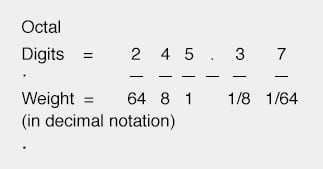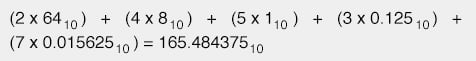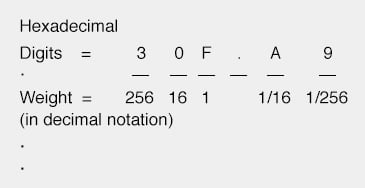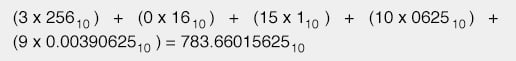Vol. Digital Circuits
Chapter 1 Numeration Systems

# Octal and Hexadecimal to Decimal Conversion

Although the prime intent of octal and hexadecimal numeration systems is for the “shorthand” representation of binary numbers in digital electronics, we sometimes have the need to convert from either of those systems to decimal form.

Of course, we could simply convert the hexadecimal or octal format to binary, then convert from binary to decimal, since we already know how to do both, but we can also convert directly.

Because octal is a base-eight numeration system, each place-weight value differs from either adjacent place by a factor of eight.

For example, the octal number 245.37 can be broken down into place values as such:The decimal value of each octal place-weight times its respective cipher multiplier can be determined as follows:The technique for converting hexadecimal notation to decimal is the same, except that each successive place-weight changes by a factor of sixteen.

Simply denote each digit’s weight, multiply each hexadecimal digit value by its respective weight (in decimal form), then add up all the decimal values to get a total.

For example, the hexadecimal number 30F.A916 can be converted like this:These basic techniques may be used to convert a numerical notation of any base into decimal form, if you know the value of that numeration system’s base.

RELATED WORKSHEETS:

#### Lessons in Electric Circuits

##### Volumes »
Published under the terms and conditions of the Design Science License